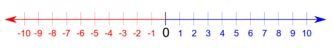## LetsPlayMaths.Com

WELCOME TO THE WORLD OF MATHEMATICS

# Class 5 Integer

Integers

Absolute value of an Integer

Comparison of integers

Addition of Positive Integers

Addition of Negative Integers

Addition of Positive and Negative Integers

Subtraction of Integers

Integer Test

Integer Worksheet

Answer Sheet

## Integers

When positive numbers, negative numbers and zero taken together are known as integers.

-4, -3, -2, -1, 0, +1, +2, +3, +4, ... are all integers.

+1, +2, +3, +4, ... known as positive integers and written as 1, 2, 3, 4, ...

-4, -3, -2, -1, ... known as negative integers.

0 is an integer but neither positive nor negative.

## Absolute value of an Integer

As we know 5 and -5 are same distance from 0. Both the integers are 5 units away from 0. The unit irrespective of the sign of the numbers is called absolute value.

Thus, the absolute value of 5 and -5 is 5. Two bars II stand for absolute value of the number written between them.

It is denoted as I 5 I = 5 and I -5 I = 5

Example 1. Find the absolute value of -8.

Solution. l -8 l = 8

Example 2. Find the absolute value of +15.

Solution. l +15 l = 15

## Comparison of integersNumber on the right is greater than the number on the left.

7 is on the right of 3, hence 7 > 3.
-2 is on the right of -9, hence -2 > -9.

## Addition of Positive Integers

When two positive integers are added, first add both the numbers and positive sigh is given for the sum. Let’s have a look at some example.

Example 1. Add +3 and +5.

Solution. (+3) + (+5) = +8.

Example 2. Add +9 and +11.

Solution. (+9) + (+11) = +20.

## Addition of Negative Integers

When two negative integers are added, first add both the numbers and negative sign is given for the sum. Let’s have a look at some example.

Example 1. Add -5 and -7.

Solution. (-5) + (-7) = -12.

Example 2. Add -25 and -15.

Solution. (-25) + (-15) = -40.

## Addition of Positive and Negative Integers

One positive and one negative number are added by subtracting them and giving the sign of the greater number to the result. Let’s have a look at some example.

Example 1. Add + 15 and -3.

Solution. (+15) + (-3) = +12.

Example 2. Add -16 and +8.

Solution. (-16) + (+8) = -8.

## Subtraction of Integers

Subtraction of integer is nothing but adding the minuend and subtrahend by changing the sign of the subtrahend. Let’s have a look at some examples.

Example 1. Subtract +12 from +18.

Solution. (+18) - (+12)
Keep minuend same, change the sign of subtrahend and then add.
(+18) + (-12)
Now, we have one positive and one negative number. We have to subtract and then give bigger number sign.
So, the result will be +6.

Integer Test- 1

Integer Test- 2

## Integer Worksheet

Integer Worksheet- 1

Integer Worksheet- 2

Integer Worksheet- 3

Integer Worksheet- 4

## Answer Sheet

Integer-AnswerDownload the pdf

Copyright © 2021 LetsPlayMaths.com. All Rights Reserved.